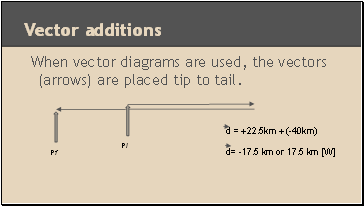# Speed and velocityPage 1

#### WATCH ALL SLIDES

Slide 1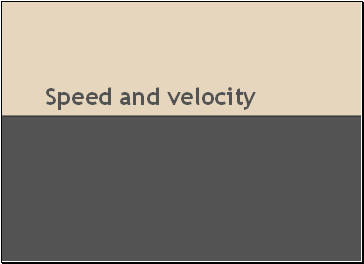Speed and velocity

Slide 2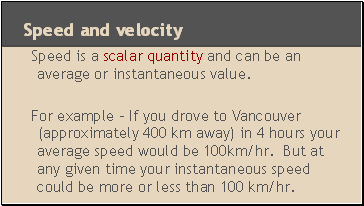## Speed and velocity

Speed is a scalar quantity and can be an average or instantaneous value.

For example - If you drove to Vancouver (approximately 400 km away) in 4 hours your average speed would be 100km/hr. But at any given time your instantaneous speed could be more or less than 100 km/hr.

Slide 3## Equations

v = d , where d = change in position (distance)

t t = time interval

Slide 4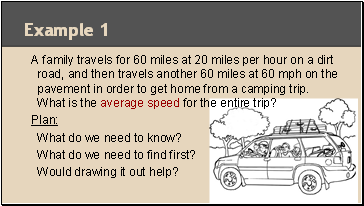Example 1

A family travels for 60 miles at 20 miles per hour on a dirt road, and then travels another 60 miles at 60 mph on the pavement in order to get home from a camping trip. What is the average speed for the entire trip?

Plan:

What do we need to know?

What do we need to find first?

Would drawing it out help?

Slide 5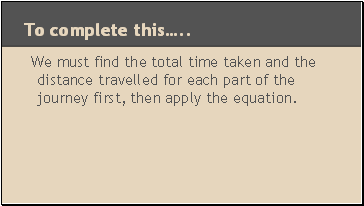To complete this…

We must find the total time taken and the distance travelled for each part of the journey first, then apply the equation.

Slide 6Example 2

A person travels for two hours at 30 miles per hour on horseback and then travels for one hour at 15 mph. What is the person’s average speed?

Slide 7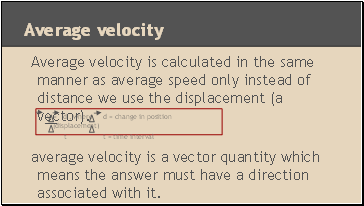## Average velocity

Average velocity is calculated in the same manner as average speed only instead of distance we use the displacement (a vector).

average velocity is a vector quantity which means the answer must have a direction associated with it.

v = d , where d = change in position (displacement)

t t = time interval

Slide 8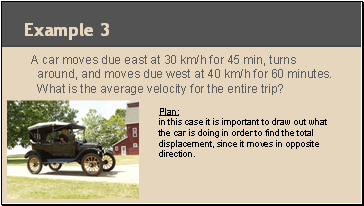Example 3

A car moves due east at 30 km/h for 45 min, turns around, and moves due west at 40 km/h for 60 minutes. What is the average velocity for the entire trip?

Plan:

in this case it is important to draw out what the car is doing in order to find the total displacement, since it moves in opposite direction.

Slide 9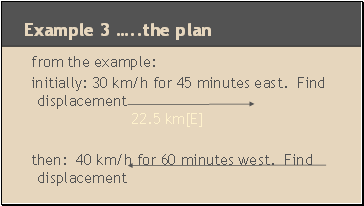from the example:

initially: 30 km/h for 45 minutes east. Find displacement 22.5 km[E]

then: 40 km/h for 60 minutes west. Find displacement

40 km [W]

Example 3 … the plan

Slide 10